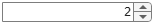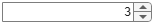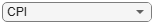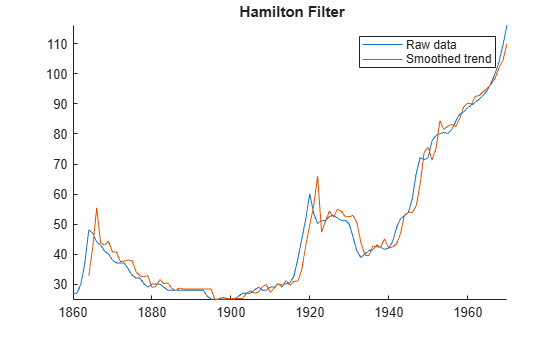# hfilter

Hamilton filter for trend and cyclical components

Since R2023a

## Syntax

``[Trend,Cyclical] = hfilter(Y)``
``[TTbl,CTbl] = hfilter(Tbl)``
``[___] = hfilter(___,Name=Value)``
``hfilter(___)``
``hfilter(ax,___)``
``````[___,h] = hfilter(___)``````

## Description

Separate one or more time series into trend and cyclical components by applying the Hamilton filter . `hfilter` optionally plots the series and trend component, with cycles removed. The plot helps you select a smoothing parameter.

In addition to the Hamilton filter, Econometrics Toolbox™ supports the Baxter-King (`bkfilter`), Christiano-Fitzgerald (`cffilter`), and Hodrick-Prescott (`hpfilter`) filters.

example

````[Trend,Cyclical] = hfilter(Y)` returns the additive trend `Trend` and cyclical `Cycilcal` components from applying the Hamilton filter to each variable (column) of the input matrix of time series data `Y`.```

example

````[TTbl,CTbl] = hfilter(Tbl)` returns the tables or timetables `TTbl` and `CTbl` containing variables for the trend and cyclical components, respectively, from applying the Hamilton filter to each variable in the input table or timetable `Tbl`. To select different variables in `Tbl` to filter, use the `DataVariables` name-value argument.```

example

````[___] = hfilter(___,Name=Value)` specifies options using one or more name-value arguments in addition to any of the input argument combinations in previous syntaxes. `hfilter` returns the output argument combination for the corresponding input arguments. For example, `hfilter(Tbl,LeadLength=4,DataVariables=1:5)` applies the Hamilton filter to the first five variables in the input table `Tbl`, and, for each selected variable, specifies the lead yt + 4 as the response variable in the filter weight regression.```

example

````hfilter(___)` plots time series variables in the input data and their respective smoothed trend components (cycles removed), computed by the Hamilton filter, on the same axes.```
````hfilter(ax,___)` plots on the axes specified by `ax` instead of the current axes (`gca`). `ax` can precede any of the input argument combinations in the previous syntaxes.```

example

``````[___,h] = hfilter(___)``` plots the specified series and their trend components, and additionally returns handles to plotted graphics objects. Use elements of `h` to modify properties of the plot after you create it.```

## Examples

collapse all

Plot the cyclical component of the US post-WWII, seasonally adjusted, quarterly, real gross national product (`GNPR`).

```load Data_GNP GNPR = Data(:,2); [trend,cyclical] = hfilter(GNPR); T = numel(trend)```
```T = 235 ```

`trend` and `cyclical` are 235-by-1 vectors containing the trend and cyclical components, respectively, resulting from applying the Hamilton filter to the series with default lead and lag lengths. The first 7 values are `NaN`s.

```plot(dates,cyclical) axis tight ylabel("Real GNP Cyclical Component")```Apply the Hamilton filter to all variables in input table variables.

Load the Schwert stock data set `Data_SchwertStock.mat`, which contains monthly returns of the NYSE index from 1871 through 2008 in `DataTimeTableMth`, among three other variables (for details, enter `Description`). Remove all missing observations from all series.

```load Data_SchwertStock TTM = rmmissing(DataTimeTableMth);```

Aggregate the monthly data in the timetable to quarterly measurements.

`TTQ = convert2quarterly(TTM);`

Apply the Hamilton filter to all variables in the quarterly timetable. Use the default lead and lag lengths.

```[TQTT,CQTT] = hfilter(TTQ); size(TQTT)```
```ans = 1×2 220 4 ```

`TQTT` and `CQTT` are 220-by-4 timetables containing the trend and cyclical components, respectively, of the series in `TTQ`. Variables in the input and output timetables correspond. By default, `hfilter` filters all variables in the input table or timetable. To select a subset of variables, set the `DataVariables` option.

The default lead and lag lengths are 4. Consequently, the first 7 rows in the output timetable are `NaN`-valued.

Remove the leading and lagging `NaN`s from the trends and display what remains.

```TQTTCut = rmmissing(TQTT); CQTTCut = rmmissing(CQTT); TQTTCut```
```TQTTCut=209×4 timetable Time Return DivYld CapGain CapGainA ___________ __________ _________ ___________ ___________ 31-Dec-1873 0.0052994 0.0037312 -0.00091529 0.00032353 31-Mar-1874 0.0028083 0.003099 -0.0024575 -0.0018408 30-Jun-1874 0.0028806 0.0041575 -0.0027131 -0.0019406 30-Sep-1874 0.0033883 0.0030027 -0.0018109 -0.0014369 31-Dec-1874 0.0053945 0.0032678 8.5274e-05 0.00027652 31-Mar-1875 0.0051083 0.0026911 0.0007776 0.00072217 30-Jun-1875 0.0035854 0.0032398 -0.00070225 -0.00041718 30-Sep-1875 0.0081389 0.0037002 0.0032879 0.0045539 31-Dec-1875 0.0052623 0.0029082 0.00081654 0.00098183 31-Mar-1876 0.0074993 0.0033872 0.0030136 0.0029454 30-Jun-1876 -0.0024581 0.0033423 -0.0052757 -0.0060788 30-Sep-1876 0.006896 0.0022414 -0.00079166 0.00056363 31-Dec-1876 0.0041519 0.0043172 0.00012738 -8.1701e-06 31-Mar-1877 0.0035486 0.0025065 -0.0013859 -0.001144 30-Jun-1877 0.0049609 0.0041852 0.00028714 0.00045375 30-Sep-1877 0.0043711 0.0038399 0.00039172 0.00027915 ⋮ ```
`CQTTCut`
```CQTTCut=209×4 timetable Time Return DivYld CapGain CapGainA ___________ __________ ___________ ___________ __________ 31-Dec-1873 0.12276 -0.0018286 0.0963 0.12583 31-Mar-1874 -0.02564 -0.0010152 -0.010496 -0.023075 30-Jun-1874 3.9596e-05 -0.0010583 -0.002827 0.0017616 30-Sep-1874 0.016762 -0.0027748 0.018478 0.021359 31-Dec-1874 -0.0067498 0.001779 -0.0028101 -0.0066786 31-Mar-1875 0.012784 0.00012197 0.010181 0.014357 30-Jun-1875 -0.011816 0.00066183 -0.02152 -0.011715 30-Sep-1875 -0.020076 -4.8708e-05 -0.014556 -0.020143 31-Dec-1875 -0.0097873 -0.00063718 -0.00081654 -0.0077777 31-Mar-1876 -0.011327 -0.0013573 -0.0057609 -0.0088029 30-Jun-1876 0.0094729 0.0010775 -0.00067668 0.0086738 30-Sep-1876 -0.061711 0.0034416 -0.059335 -0.061062 31-Dec-1876 0.0017068 0.0025794 -0.0070239 -0.0010298 31-Mar-1877 -0.040981 0.00309 -0.050659 -0.041885 30-Jun-1877 -0.09557 0.0024379 -0.072017 -0.097686 30-Sep-1877 0.059143 0.0020562 0.060833 0.057339 ⋮ ```

To compare outputs between different tabular inputs, apply the Hamilton filter to all variables in the table of monthly data `DataTableMth` and the timetable of monthly data `TTM`.

```% Table input of daily data DTM = rmmissing(DataTableMth); [TMDT,CMDT] = hfilter(DTM); TMDT = rmmissing(TMDT); CMDT = rmmissing(CMDT); size(TMDT)```
```ans = 1×2 645 4 ```
`tail(TMDT)`
``` Return DivYld CapGain CapGainA _________ _________ __________ __________ May1925 0.0076851 0.0035499 0.0033316 0.0032399 Jun1925 0.007946 0.0055856 0.0038195 0.0037338 Jul1925 0.010239 0.0044464 0.0063502 0.0063067 Aug1925 0.0080799 0.0035821 0.003389 0.0037078 Sep1925 0.0076608 0.0055354 0.0037118 0.003575 Oct1925 0.0081879 0.004496 0.0042474 0.0041497 Nov1925 0.005312 0.0034493 0.00060642 0.00071388 Dec1925 0.009001 0.0049537 0.0052133 0.0048893 ```
`tail(CMDT)`
``` Return DivYld CapGain CapGainA __________ ___________ __________ __________ May1925 0.058501 -0.0010668 0.060371 0.060463 Jun1925 -0.0047057 -0.00012725 -0.0060375 -0.0059518 Jul1925 0.0086164 0.00076594 0.0072928 0.0073363 Aug1925 0.040128 -0.00087019 0.042107 0.041788 Sep1925 0.0091173 -0.00023334 0.0077642 0.007901 Oct1925 0.060009 0.00014365 0.05931 0.059407 Nov1925 -0.013777 -0.00063483 -0.011885 -0.011993 Dec1925 0.04059 0.0021392 0.037285 0.037609 ```
```% Timetable input of daily data [TMTT,CMTT] = hfilter(TTM); TMTT = rmmissing(TMTT); CMTT = rmmissing(CMTT); size(TMTT)```
```ans = 1×2 645 4 ```
`tail(TMTT)`
``` Time Return DivYld CapGain CapGainA ___________ _________ _________ __________ __________ 01-May-1925 0.0076851 0.0035499 0.0033316 0.0032399 01-Jun-1925 0.007946 0.0055856 0.0038195 0.0037338 01-Jul-1925 0.010239 0.0044464 0.0063502 0.0063067 01-Aug-1925 0.0080799 0.0035821 0.003389 0.0037078 01-Sep-1925 0.0076608 0.0055354 0.0037118 0.003575 01-Oct-1925 0.0081879 0.004496 0.0042474 0.0041497 01-Nov-1925 0.005312 0.0034493 0.00060642 0.00071388 01-Dec-1925 0.009001 0.0049537 0.0052133 0.0048893 ```
`tail(CMTT)`
``` Time Return DivYld CapGain CapGainA ___________ __________ ___________ __________ __________ 01-May-1925 0.058501 -0.0010668 0.060371 0.060463 01-Jun-1925 -0.0047057 -0.00012725 -0.0060375 -0.0059518 01-Jul-1925 0.0086164 0.00076594 0.0072928 0.0073363 01-Aug-1925 0.040128 -0.00087019 0.042107 0.041788 01-Sep-1925 0.0091173 -0.00023334 0.0077642 0.007901 01-Oct-1925 0.060009 0.00014365 0.05931 0.059407 01-Nov-1925 -0.013777 -0.00063483 -0.011885 -0.011993 01-Dec-1925 0.04059 0.0021392 0.037285 0.037609 ```

Because the data is disaggregated, the outputs of the daily data have more rows than from the quarterly data. The filter results of the daily inputs are equal among the corresponding outputs, but hfilter returns tables of results, instead of timetables, when you supply data in a table.

Load the Nelson-Plosser macroeconomic data set `Data_NelsonPlosser.mat`, which contains series measured yearly in the timetable `DataTimeTable`.

`load Data_NelsonPlosser`

Apply the Hamilton filter to the real and nominal GNP series, `GNPR` and `GNPN`, respectively. Set the lead and lag lengths 2 years and 1 year, respectively. Plot the trend component with each series.

```hfilter(DataTimeTable,DataVariables=["GNPR" "GNPN"], ... LeadLength=2,LagLength=3);```Experiment with filter parameter values by adjusting the interactive controls.

```varnames = string(DataTimeTable.Properties.VariableNames); lead =2; % LeadLength lag =3; % LagLength vn =varnames(8); % DataVariables figure [TTbl,CTbl,h] = hfilter(DataTimeTable,DataVariables=vn, ... LeadLength=lead,LagLength=lag);```## Input Arguments

collapse all

Time series data, specified as a `numObs`-by-`numVars` numeric matrix. Each column of `Y` corresponds to a variable, and each row corresponds to an observation.

Data Types: `double`

Time series data, specified as a table or timetable with `numObs` rows. Each row of `Tbl` is an observation.

Specify `numVars` variables to filter by using the `DataVariables` argument. The selected variables must be numeric.

Axes on which to plot, specified as an `Axes` object.

By default, `hfilter` plots to the current axes (`gca`).

Note

`hfilter` removes, from the specified data, all rows containing at least one missing observation, represented by a `NaN` value.

### Name-Value Arguments

Specify optional pairs of arguments as `Name1=Value1,...,NameN=ValueN`, where `Name` is the argument name and `Value` is the corresponding value. Name-value arguments must appear after other arguments, but the order of the pairs does not matter.

Before R2021a, use commas to separate each name and value, and enclose `Name` in quotes.

Example: `hfilter(Tbl,LeadLength=4,DataVariables=1:5)` applies the Hamilton filter to the first five variables in the input table `Tbl`, and, for each selected variable, specifies the lead yt + 4 as the response variable in the filter weight regression.

Filter weight regression response variable lead h, in units of data periodicity, specified as a positive integer or vector of positive integers. That is, the response variable in the regression is yt+h.

For a scalar, `hfilter` applies `LagLength` to all input series.

For a vector, `hfilter` applies `LagLength(j)` to series `j` in the input data.

The default is `8` quarters, suggested in .

Example: `LeadLength=[4 8]` specifies that the response variable of the filter weight regression for the first series in the input data is yt+4 and the response variable for the second input series is yt+8.

Data Types: `double`

Number of consecutive lags p of predictor variables yt, yt–1, …, yt+p–1 in the filter weight regression, in units of data periodicity, specified as a positive integer or vector of positive integers. `hfilter` includes lags 0 through p – 1.

For a scalar, `hfilter` applies `LagLength` to all input series.

For a vector, `hfilter` applies `LagLength(j)` to series `j` in the input data.

The default is `4` quarters, suggested in .

Example: `LagLength=[4 8]` specifies lags 0 through `3` as predictors for the regression when filtering the first series of the input data and lags 0 through `8` as predictors for the regression when filtering the second series.

Data Types: `double`

Variables in `Tbl` that `hfilter` filters, specified as a string vector or cell vector of character vectors containing variable names in `Tbl.Properties.VariableNames`, or an integer or logical vector representing the indices of names. The selected variables must be numeric.

Example: `DataVariables=["GDP" "CPI"]`

Example: `DataVariables=[true true false false]` or `DataVariables=[1 2]` selects the first and second table variables.

Data Types: `double` | `logical` | `char` | `cell` | `string`

## Output Arguments

collapse all

Smoothed trend component τt of each series in the data, returned as a `numObs`-by-`numVars` numeric matrix. `hfilter` returns `Trend` when you supply the input `Y`.

The first `LeadLength` + `LagLength` – 1 values are `NaN`.

Cyclical component ct of each series in the data, returned as a `numObs`-by-`numVars` numeric matrix. `hfilter` returns `Cyclical` when you supply the input `Y`.

The first `LeadLength` + `LagLength` – 1 values are `NaN`.

Smoothed trend component τt of each specified series, returned as a `numObs`-by-`numVars` table or timetable, the same data type as `Tbl`. `hfilter` returns `TTbl` when you supply the input `Tbl`.

For each selected series and corresponding `LeadLength` and `LagLength`, the first `LeadLength` + `LagLength` – 1 values are `NaN`.

Cyclical component ct of each specified series, returned as a `numObs`-by-`numVars` table or timetable, the same data type as `Tbl`. `hfilter` returns `CTbl` when you supply the input `Tbl`.

For each selected series and corresponding `LeadLength` and `LagLength`, the first `LeadLength` + `LagLength` – 1 values are `NaN`.

Handles to plotted graphics objects, returned as a vector of graphics objects. `hfilter` plots the data and trend only when you return no outputs or you return `h`.

`h` contains unique plot identifiers, which you can use to query or modify properties of the plot.

collapse all

### Hamilton Filter

The Hamilton filter separates a time series yt into a trend component τt (`Trend` and `TTbl`) and cyclical component ct (`Cyclical` and `CTbl`) such that yt = τt + ct.

The Hamilton filter performs an autoregression of yt+h on a constant and yt, yt–1, …, ytp+1, where h is the value of the `LeadLength` name-value argument and p is the value of the `LagLength` name-value argument. The filter identifies the trend and cyclical components of yt at time t + h with the expected value of yt+h and the forecast error, respectively . The decomposition is based on a definition of business cycle in .

## Tips

• Regarding a setting for the `LeadLength` name-value argument, Hamilton  states "If we are interested in business cycles, a 2-year horizon should be the standard benchmark." Regarding a setting for the `LagLength` name-value argument, the article states "One might be tempted to use a richer model to forecast yt+h, such as using more than 4 lags, or even a nonlinear relation. However, such refinements are completely unnecessary for the goal of extracting a stationary (cyclical) component, and have the significant drawback that the more parameters we try to estimate by regression, the more the small-sample results are likely to differ from the asymptotic predictions."

 Beveridge, Stephen, and Charles R. Nelson. "A New Approach to Decomposition of Economic Time Series into Permanent and Transitory Components with Particular Attention to Measurement of the 'Business Cycle.'" Journal of Monetary Economics 7 (January 1981): 151–74. https://doi.org/10.1016/0304-3932(81)90040-4.

 Hamilton, James D. "Why You Should Never Use the Hodrick-Prescott Filter." The Review of Economics and Statistics 100 (December 2018): 831–43. https://doi.org/10.1162/rest_a_00706.